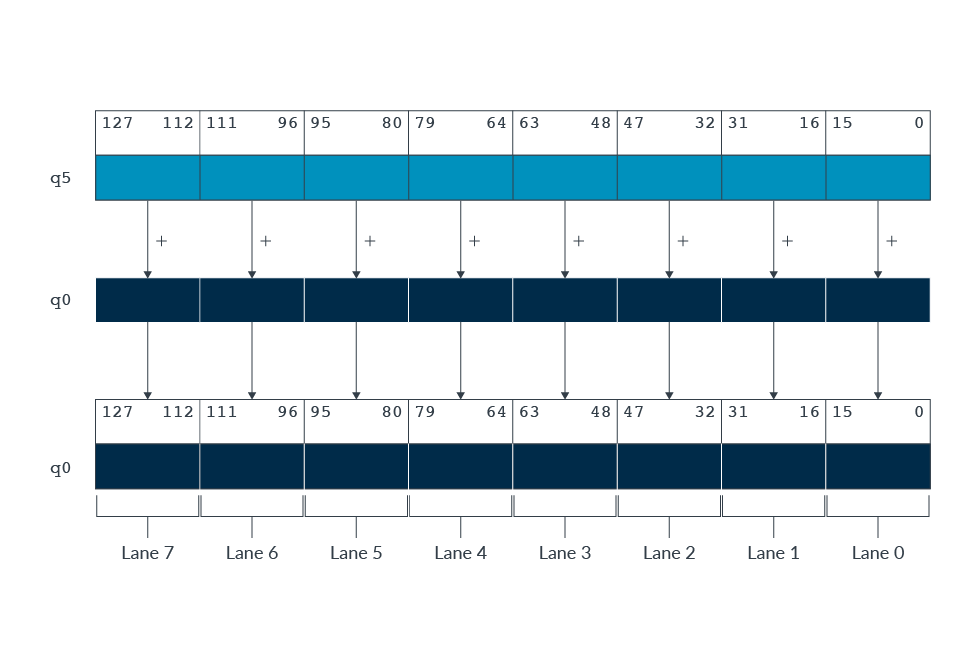## Helium registers

##### Registers, vectors, lanes, and elements

The Helium registers contain vectors of elements of the same data type. The same element position in the input and output registers is referred to as a lane.

Usually each Helium instruction results in noperations, where nis the number of lanes that the input vectors are divided into. Each operation is contained within the lane.

The number of lanes in a Helium vector depends on the size of the vector and the data elements in the vector.

A 128-bit Helium vector can contain the following element sizes:

• Two 64-bit integers
• Four 32-bit integers or single precision float
• Eight 16-bit integers or half precision float
• Sixteen 8-bit integers

Elements in a vector are ordered from the least significant bit to the most significant bit. That is, element 0 uses the least significant bits of the register. Let’s look at an example of a Helium instruction. The instruction `VADD.16 q0, q0, q5` performs a parallel addition of eight lanes of 16-bit (8 x 16 = 128) integer elements from vectors in `q5` and `q0`, storing the result in `q0`. This can be seen in the following diagram:Helium instructions use a mix of vector and scalar operands, including:

• Vector by vector to vector
• Vector by scalar to vector
• Vector by vector to scalar

For example, multiplication, this can be seen in Vector instruction example. You can find more examples in the Armv8-M Architecture Reference Manual

##### Data types

When programming for Helium in C or C++, different data types let you declare vectors of different sizes. To use these data types, we must add the library `arm_mve.h` to the program. This header file provides data types that look like the following:

• Sixteen 8-bit elements = `int8x16, uint8x16, float8x16`
• Eight 16-bit elements = `int16x8, uint16x8, float16x8`
• Four 32-bit elements = `int32x4, uint32x4, float32x4`
• Two 64-bit elements = `int64x2, uint64x2, float64x2`
##### Predication register

Predication lets you selectively perform mathematic operations on lanes in a vector. The predication mask specifies which lanes are processed, by setting bits to true (1) or false (0). The predication status and control register, `VPR.P0`, contains this predication mask. We explain this in Helium instructions.